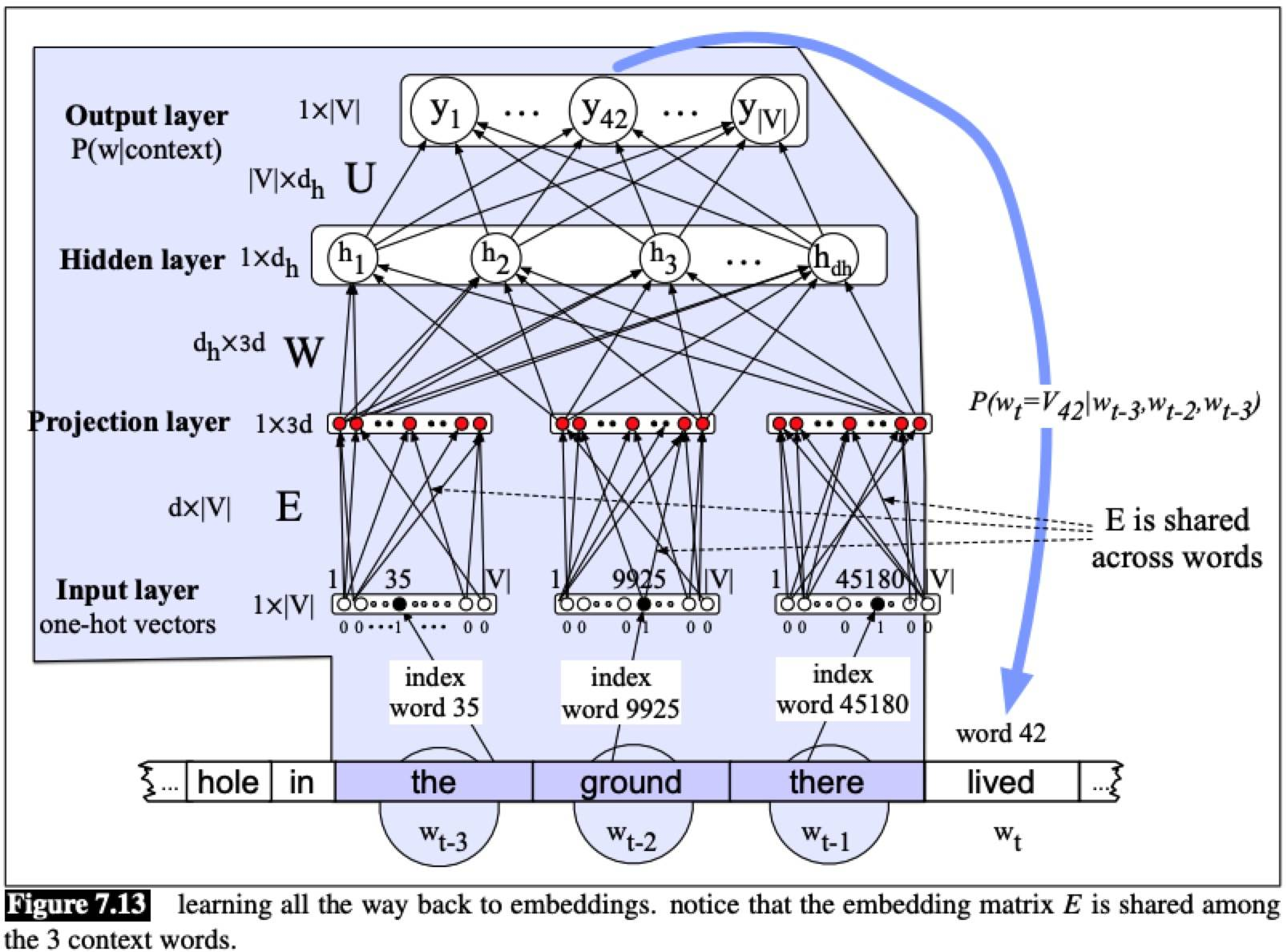# Neural Networks and Neural Language Models Note (SLP Ch07)

## Units

In practice, the sigmoid is not commonly used as an activation function. A better one is tanh function ranges from -1 to 1: $y = \frac{e^z - e^{-z}}{e^z + e^{-z}}$

The most commonly used is the rectified linear unit, also called ReLU: y = max(x, 0)​

In the sigmoid or tanh functions, very high values of z result in values of y that are saturated, extremely close to 1, which causes problems for learning.

• Rectifiers don’t have this problem, since the output of values close to 1 also approaches 1 in a nice gentle linear way.
• By contrast, the tanh function has the nice properties of being smoothly differentiable and mapping outlier values toward the mean.

## The XOR problem

It’s not possible to build a perceptron to compute logical XOR. The intuition behind this important result relies on understanding that a perceptron is a linear classifier. XOR is not a linearly separable function.

### The solution: neural networks

The hidden layer is forming a representation for the input. The solution need a network with non-linear activation functions.

A network with simple linear (perceptron) units cannot solve the XOR problem, because a network formed by many layers of purely linear units can always be reduced (shown to be computationally identical to) a single layer of linear units with appropriate weights.

## Feed-Forward Neural Networks

A feed-forward network is a multilayer network in which the units are connected with no cycles.

h forms a representation of the input, out layer is to take this new representation to compute a final output.

Softmax was exactly what is used to create a probability distribution from a vector of real-valued numbers. It is called normalizing.

A neural network is like logistic regression, but

• with many layers, a DNN is like layer after layer of LR classifiers
• DNN forming features automatically

The activation functions g(·) are generally different at the final layer.

• softmax for multinomial classification or sigmoid for binary classification
• RELU or tanh might be the activation function at the internal layers

## Training Neural Nets

In LR, for each observation we could directly compute the derivative of the loss function with respect to an individual w or b. But for NN, with millions of parameters in many layers, it’s much harder to compute the partial derivative of some weight in layer 1 when the loss is attached to some much later layer.

The algorithm we use is called error back-propagation or reverse differentiation.

### Loss function

Loss function here is being used as a binary classifier, with the sigmoid at the final layer.

The cross-entropy loss is simply the log probability of the correct class, called negative log likelihood loss:

With softmax formula and K classes:

### Backward differentiation on computation graphs

We need to know the derivatives of all the functions in the graph:

• sigmoid: $\frac {d\sigma(z)}{dz} = \sigma(z) (1-z)$
• tanh: $\frac{d \tanh(z)}{dz} = 1 - \tanh^2(z)$
• ReLU: $\frac{d ReLU(z)}{dz} = 0\ for\ x<0;\ 1\ for\ x≥0$

Here is a simple example from CS231n.

### More details on learning

• For LR, we can initialize gradient descent with all the weights and biases having the value 0. In DNN, we need to initialize the weights with small random numbers, also helpful to normalize the input values to have 0 mean and unit variance.

• Dropout is used to prevent overfitting: randomly dropping some units and their connections from the network during training.

• Hyperparameter tuning includes the learning rate, the minibatch size, the model architecture, how to regularize and so on. Gradient descent itself also has many architectural variants such as Adam.

## Neural Language Models

Compared with N-gram models:

• do not need smoothing
• higher predictive accuracy
• underlie many other task models (MT, Dialog, LG)

### Embeddings### Training the neural language model

At each word wt, the cross-entropy (negative log likelihood) loss is: